Maria's Math News, March 2015
 Hello! I have finally been able to edit & upload some new videos. They are about the square root, the Pythagorean Theorem, and statistics. More will be coming!~Maria Math Mammoth news Videos (grades 6-8) Number bonds (grades 1-3) Puzzle corner was too easy! (grades 1-4) Rolling Thrones: An Exponent Game - The Results (grades 5-8)

## 1. Math Mammoth newsThis is for you if you'd like to start using Math Mammoth Grade 7-B now. Like I have mentioned in the past, since 7-B is not available yet, we are including the lessons from the proportions chapter of 7-B in the book Math Mammoth Ratios, Proportions & Problem Solving.

You can get that book to "keep you going" for now. If you've purchased it (either separately or as part of a Blue series bundle) in the past, you can get the updated version for free. Just contact me.

If you need to purchase it for this purpose, I will reimburse you for that amount from the price of 7-B when it comes available.

## 2. Videos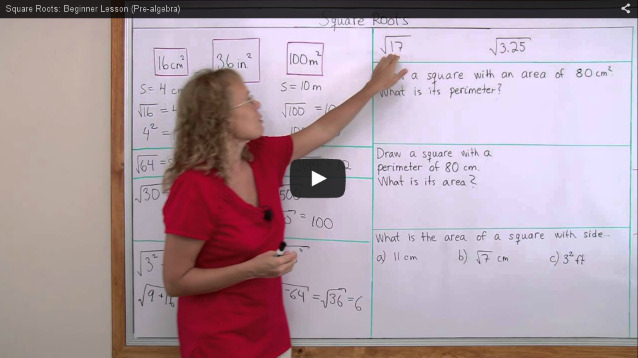I have finally been able to edit & upload some new videos. These are the ones I've published within the last few weeks:

More will be coming soon!

## 3. Number bonds

Someone sent me a question on NUMBER BONDS:
"I was wondering if you use number bonds, like in Singapore Math, for learning math facts? Or if you use any similar pictorial approach to memorizing math facts?"
Good question!

Math Mammoth uses two main approaches to teach addition & subtraction facts:

(1) mental math strategies

and

(2) fact families (the same concept as number bonds).

An example of (1) would be a trick with 9. For example, to add 9 + 5, child is instructed to think that 9 wants to become 10, so 5 generously gives "one" to 9, becoming 4. So the addition 9 + 5 is changed into 10 + 4 (which is easy).

Another example — a very important strategy — is to use addition to solve subtractions. For example, with the problem 9 − 4 = ___, the child should think, "4 plus what number makes 9?" This is especially important with those facts where the sum is between 10 and 18. For example, to solve 15 − 7, the child needs to remember the ADDITION fact 7 + 8 = 15 and use that, instead of memorizing the subtraction facts separately.

That is also why I use fact families (or number bonds as they are also called). The idea is to associate/memorize the numbers 7, 8, and 15 — or in other words memorize 7 + 8 = 15 and understand conceptually that that gives us another addition fact (8 + 7 = 15) and two subtraction facts (15 − 8 = 7 and 15 − 7 = 8).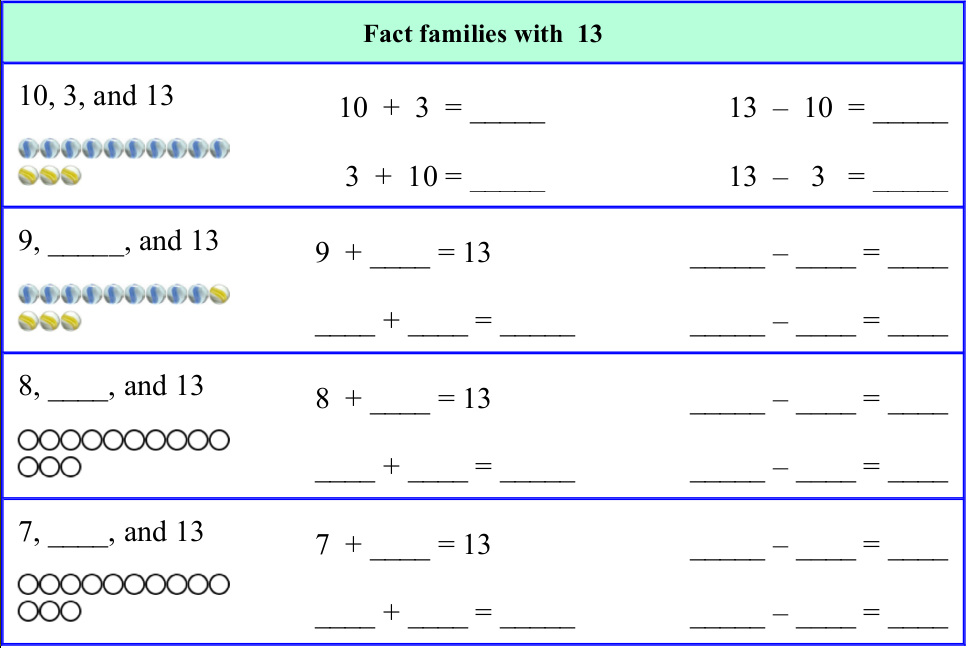You can also check these sample pages (PDF) from my books Subtraction 1 and Add & Subtract 2-A:

• Subtraction_1_Fact_Families.pdf
• AS2A_Number_Rainbows.pdf
• AS2A_Fact_Families_13.pdf

• ## 4. Puzzle corner was too easy!

One of my customers mentioned that this particular puzzle corner from Math Mammoth Grade 1-A was too easy for her son:

I admit, it's kind of easy. But, like I told her, I'm sure some children enjoy having an easier puzzle corner in between the others because several people have mentioned they are challenging to their children.

But, you can make it to be more challenging very easily: simply add a number to each equation... and possibly also make (some of) the numbers bigger. For example:
 Originally: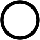− 4 = 5 Change it to:− 4 − 2 = 5 Originally: 5 −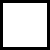= 4 Change it to: 10 − 5 −= 4 Originally: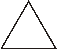+ 4 = 5 Change it to: 2 ++ 4 = 11

And similarly the others. He can even enjoy the challenge of changing the problems himself... and then solving them!

## 5. Rolling Thrones: An Exponent Game

Simplify With Me blog did an experiment to see if playing games in the classroom was worthwhile. She had one class period play a game about exponents and lectured and gave a worksheet to the other class period. Read her post to see her results!

=> Rolling Thrones Exponent Game Results

 That's it this time! I hope you found something helpful.Feel free to forward this issue to a friend/colleague! Subscribe here. Till next month, Maria Miller

--